# Setup for 5 Player a Game of Food Chain Magnate

Originally generated on 1/10/2019 11:48:11 PM
A direct link to this setup is http://www.VentersConsulting.com/BoardgameHelpers/FoodChainMagnateGenerator.aspx?LoadGame=\$2a\$04\$4FuJCaQd3Wfk8OHKyke2Au

## Player Setup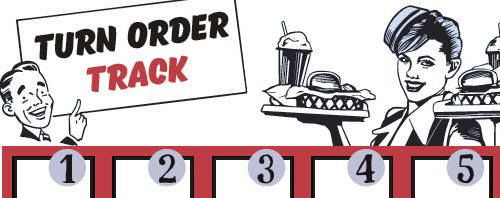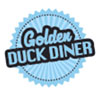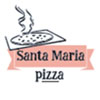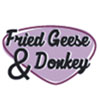## Other Setup

Bank starts with \$250.00
Number of 1x Employee cards used = 3

## Map (Broken Roads)

Click here to view Map Tile Lettering Key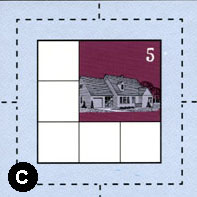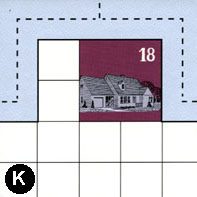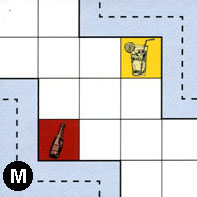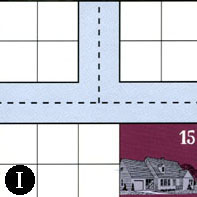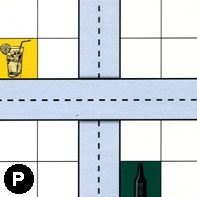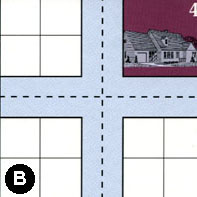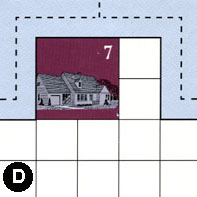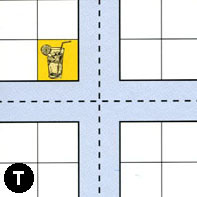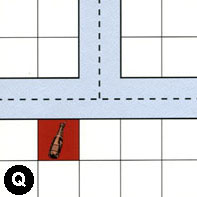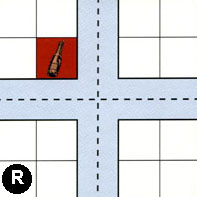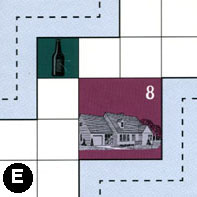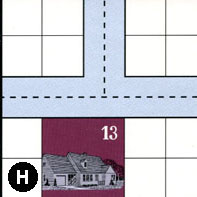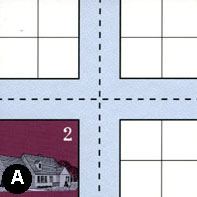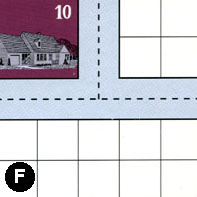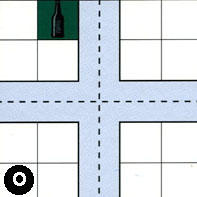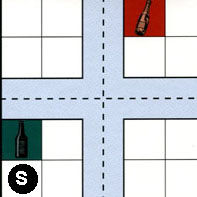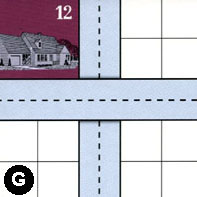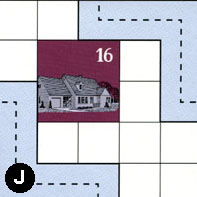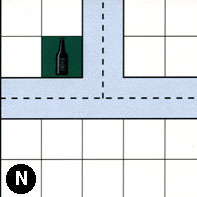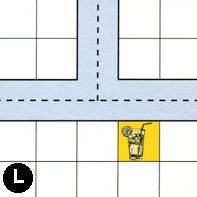### Map Stats

Map Option = Broken Roads
Total Number of Tiles = 20
Total Number of Starting Houses = 11
Total Number of Beer Spots = 5
Total Number of Soda Spots = 4
Total Number of Lemonade Spots = 4
Total Number of Drink Spots = 13
Number of independent path systems = 4
Number of independent neighborhoods = 17

Path System 1
Size = Medium
Number of Tiles = 5
Number of Paths = 17
Contains Loop(s) = No
Number of Starting Houses = 4
Starting Houses Pct of Map (%) = 36
Number of Beer Spots = 1
Number of Soda Spots = 1
Number of Lemonade Spots = 0
Number of Drink Spots = 2
Drink Spots Pct of Map (%) = 15
Tile Ids = B, C, E, K, M
Paths = CWE, BWN, BNE, EWN, BWE, CWN, CNE, CES, KWN, KNE, MWS, KWE, CWS, BWS, BNS, BES, CNS What do these mean?
Starting House Ids = 0, 4, 5, 18
Path System 2
Size = Medium
Number of Tiles = 10
Number of Paths = 28
Contains Loop(s) = No
Number of Starting Houses = 7
Starting Houses Pct of Map (%) = 64
Number of Beer Spots = 2
Number of Soda Spots = 0
Number of Lemonade Spots = 2
Number of Drink Spots = 4
Drink Spots Pct of Map (%) = 31
Tile Ids = A, D, G, H, I, J, M, N, P, T
Paths = IWN, MEN, TWE, AWN, HWN, DWE, DWN, TWN, ANE, AWE, HNE, GWE, HWE, DNE, TNE, TES, TWS, ANS, JEN, NWE, NWN, NNE, AWS, AES, TNS, IWE, PNS, INE What do these mean?
Starting House Ids = 0, 2, 7, 12, 13, 15, 16
Path System 3
Size = Medium
Number of Tiles = 6
Number of Paths = 22
Contains Loop(s) = No
Number of Starting Houses = 2
Starting Houses Pct of Map (%) = 18
Number of Beer Spots = 1
Number of Soda Spots = 2
Number of Lemonade Spots = 2
Number of Drink Spots = 5
Drink Spots Pct of Map (%) = 38
Tile Ids = F, L, O, P, Q, R
Paths = PWE, RWE, OWS, OWN, LWN, LNE, LWE, ONE, FWN, QWE, QWN, RWN, ONS, OES, FNE, FWE, QNE, RNE, RES, RWS, RNS, OWE What do these mean?
Starting House Ids = 0, 10
Path System 4
Size = Medium
Number of Tiles = 4
Number of Paths = 9
Contains Loop(s) = No
Number of Starting Houses = 5
Starting Houses Pct of Map (%) = 45
Number of Beer Spots = 1
Number of Soda Spots = 1
Number of Lemonade Spots = 0
Number of Drink Spots = 2
Drink Spots Pct of Map (%) = 15
Tile Ids = E, G, J, S
Paths = SWS, SWN, EES, SNE, GNS, JWS, SWE, SES, SNS What do these mean?
Starting House Ids = 0, 8, 12, 13, 16

Neighborhood 1
Total Size = Huge
Number of Total Spaces = 100
Number of Empty Spaces (for new houses & gardens) = 76
Number of Beer Spots = 1
Number of Soda Spots = 2
Number of Lemonade Spots = 1
Number of Drink Spots = 4
Number of Starting Houses = 5
Starting Houses Pct of Map (%) = 45
Tile Ids = B, D, E, G, H, I, K, M, S, T
Starting House Ids = 4, 7, 8, 13, 18
Neighborhood 2
Total Size = Medium
Number of Total Spaces = 8
Number of Empty Spaces (for new houses & gardens) = 8
Number of Beer Spots = 0
Number of Soda Spots = 0
Number of Lemonade Spots = 0
Number of Drink Spots = 0
Number of Starting Houses = 0
Starting Houses Pct of Map (%) = 0
Tile Ids = I, P
Neighborhood 3
Total Size = Small
Number of Total Spaces = 4
Number of Empty Spaces (for new houses & gardens) = 3
Number of Beer Spots = 1
Number of Soda Spots = 0
Number of Lemonade Spots = 0
Number of Drink Spots = 1
Number of Starting Houses = 0
Starting Houses Pct of Map (%) = 0
Tile Ids = P
Neighborhood 4
Total Size = Medium
Number of Total Spaces = 9
Number of Empty Spaces (for new houses & gardens) = 5
Number of Beer Spots = 0
Number of Soda Spots = 0
Number of Lemonade Spots = 0
Number of Drink Spots = 0
Number of Starting Houses = 1
Starting Houses Pct of Map (%) = 9
Tile Ids = C
Starting House Ids = 5
Neighborhood 5
Total Size = Huge
Number of Total Spaces = 90
Number of Empty Spaces (for new houses & gardens) = 82
Number of Beer Spots = 1
Number of Soda Spots = 1
Number of Lemonade Spots = 2
Number of Drink Spots = 4
Number of Starting Houses = 1
Starting Houses Pct of Map (%) = 9
Tile Ids = A, F, I, L, N, O, P, Q, R, T
Starting House Ids = 15
Neighborhood 6
Total Size = Medium
Number of Total Spaces = 8
Number of Empty Spaces (for new houses & gardens) = 8
Number of Beer Spots = 0
Number of Soda Spots = 0
Number of Lemonade Spots = 0
Number of Drink Spots = 0
Number of Starting Houses = 0
Starting Houses Pct of Map (%) = 0
Tile Ids = P, R
Neighborhood 7
Total Size = Small
Number of Total Spaces = 4
Number of Empty Spaces (for new houses & gardens) = 4
Number of Beer Spots = 0
Number of Soda Spots = 0
Number of Lemonade Spots = 0
Number of Drink Spots = 0
Number of Starting Houses = 0
Starting Houses Pct of Map (%) = 0
Tile Ids = B
Neighborhood 8
Total Size = Small
Number of Total Spaces = 4
Number of Empty Spaces (for new houses & gardens) = 4
Number of Beer Spots = 0
Number of Soda Spots = 0
Number of Lemonade Spots = 0
Number of Drink Spots = 0
Number of Starting Houses = 0
Starting Houses Pct of Map (%) = 0
Tile Ids = B
Neighborhood 9
Total Size = Medium
Number of Total Spaces = 12
Number of Empty Spaces (for new houses & gardens) = 11
Number of Beer Spots = 0
Number of Soda Spots = 0
Number of Lemonade Spots = 1
Number of Drink Spots = 1
Number of Starting Houses = 0
Starting Houses Pct of Map (%) = 0
Tile Ids = A, H, T
Neighborhood 10
Total Size = Medium
Number of Total Spaces = 16
Number of Empty Spaces (for new houses & gardens) = 11
Number of Beer Spots = 0
Number of Soda Spots = 1
Number of Lemonade Spots = 0
Number of Drink Spots = 1
Number of Starting Houses = 1
Starting Houses Pct of Map (%) = 9
Tile Ids = F, O, Q, R
Starting House Ids = 10
Neighborhood 11
Total Size = Medium
Number of Total Spaces = 8
Number of Empty Spaces (for new houses & gardens) = 8
Number of Beer Spots = 0
Number of Soda Spots = 0
Number of Lemonade Spots = 0
Number of Drink Spots = 0
Number of Starting Houses = 0
Starting Houses Pct of Map (%) = 0
Tile Ids = O, R
Neighborhood 12
Total Size = Large
Number of Total Spaces = 31
Number of Empty Spaces (for new houses & gardens) = 23
Number of Beer Spots = 0
Number of Soda Spots = 0
Number of Lemonade Spots = 0
Number of Drink Spots = 0
Number of Starting Houses = 2
Starting Houses Pct of Map (%) = 18
Tile Ids = A, G, H, J, N
Starting House Ids = 2, 16
Neighborhood 13
Total Size = Medium
Number of Total Spaces = 8
Number of Empty Spaces (for new houses & gardens) = 7
Number of Beer Spots = 1
Number of Soda Spots = 0
Number of Lemonade Spots = 0
Number of Drink Spots = 1
Number of Starting Houses = 0
Starting Houses Pct of Map (%) = 0
Tile Ids = L, O
Neighborhood 14
Total Size = Small
Number of Total Spaces = 4
Number of Empty Spaces (for new houses & gardens) = 3
Number of Beer Spots = 1
Number of Soda Spots = 0
Number of Lemonade Spots = 0
Number of Drink Spots = 1
Number of Starting Houses = 0
Starting Houses Pct of Map (%) = 0
Tile Ids = S
Neighborhood 15
Total Size = Medium
Number of Total Spaces = 8
Number of Empty Spaces (for new houses & gardens) = 4
Number of Beer Spots = 0
Number of Soda Spots = 0
Number of Lemonade Spots = 0
Number of Drink Spots = 0
Number of Starting Houses = 1
Starting Houses Pct of Map (%) = 9
Tile Ids = G, S
Starting House Ids = 12
Neighborhood 16
Total Size = Small
Number of Total Spaces = 4
Number of Empty Spaces (for new houses & gardens) = 4
Number of Beer Spots = 0
Number of Soda Spots = 0
Number of Lemonade Spots = 0
Number of Drink Spots = 0
Number of Starting Houses = 0
Starting Houses Pct of Map (%) = 0
Tile Ids = G
Neighborhood 17
Total Size = Small
Number of Total Spaces = 4
Number of Empty Spaces (for new houses & gardens) = 4
Number of Beer Spots = 0
Number of Soda Spots = 0
Number of Lemonade Spots = 0
Number of Drink Spots = 0
Number of Starting Houses = 0
Starting Houses Pct of Map (%) = 0
Tile Ids = L

v8.3.2